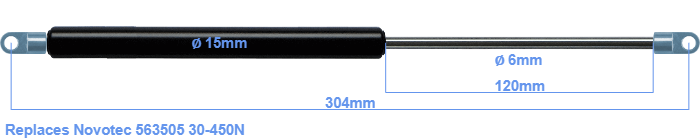# Replacement for Novotec 563505 30-450N

\$28.94

Replacement gas spring for the Novotec 563505 30-450 Newton. The eye has a thickness of 3mm and a hole diameter of 6.1mm. Brand: HAHN, a Stabilus company.

Please note:
The eye with the thickness of 3mm of this replacement gas spring can be shorter than the original. Note that this part of the eye doesn't get in the way.
 Force Choose an option30 Newton40 Newton50 Newton60 Newton80 Newton100 Newton120 Newton140 Newton150 Newton160 Newton180 Newton200 Newton220 Newton240 Newton250 Newton260 Newton280 Newton300 Newton320 Newton340 Newton350 Newton360 Newton380 Newton400 Newton420 Newton440 Newton450 NewtonClearThe diameter of the cylinder of this gas spring is 15 millimeters. The rod has a diameter of 6 mm. The stroke (the retracting part) has a length of 120 mm. The total length of this replacement gas spring is 304 mm. This is the distance between the centers of the mounting parts. The length from thread to thread (exlcuding the mounting parts) is 272 millimeter. The force of this replacement is 30-450 Newton. This is not an original gas spring from Novotec, but a so called replacement gas spring. This gas spring was produced by Stabilus subsidiary HAHN. Nevertheless, this gas spring can be used as replacement gas spring, because the dimensions and forces are almost equal.